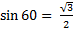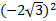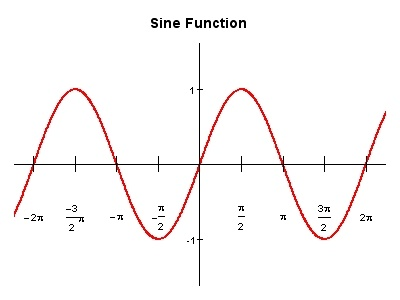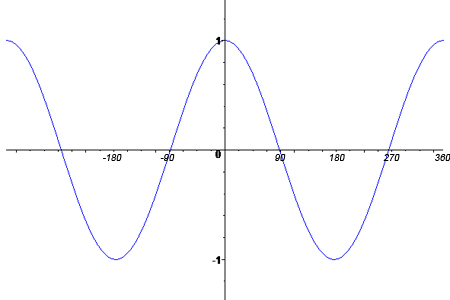# Even and Odd Trig Functions

All functions, including trig functions, can be described as being even, odd, or neither. A function is odd if and only if f(-x) = - f(x) and is symmetric with respect to the origin. A function is even if and only if f(-x) = f(x) and is symmetric to the y axis. It is helpful to know if a function is odd or even when you are trying to simplify an expression when the variable inside the trigonometric function is negative.

 sin( -x ) = - sin x csc ( -x ) = - csc x cos ( -x ) = cos x sec (-x ) = sec x tan ( -x ) = - tan x tan ( -x ) = - cot x

Example 1: find the value of (4 · sin (-60))2

= (-4 · sin (60))2     sin(-x) = - sin x

=== 12

Example 2: Determine if the following function is odd or even

f(x) = x3 sin x

Find f(-x)     f(-x) = -(-x)3sin (x)     replacing x with -x and sin (-x) = - sin x

f(-x) = x3 sin x

f(x) = f(-x) therefore the function is even.

Example 3: Determine if the graph is odd or even.The graph is symmetric with respect to the origin therefore it is on odd function.

Cosine FunctionThe graph is symmetric to the y- axis therefore it is an even function.

The majority of functions are neither odd nor even, however, sine and tangent are odd functions and cosine is an even function. This can be important information when identifying graphs.

 Related Links: Math Trigonometry Power Reduction Formula Product to Sum and Sum to Product Formulas

To link to this Even and Odd Trig Functions page, copy the following code to your site: